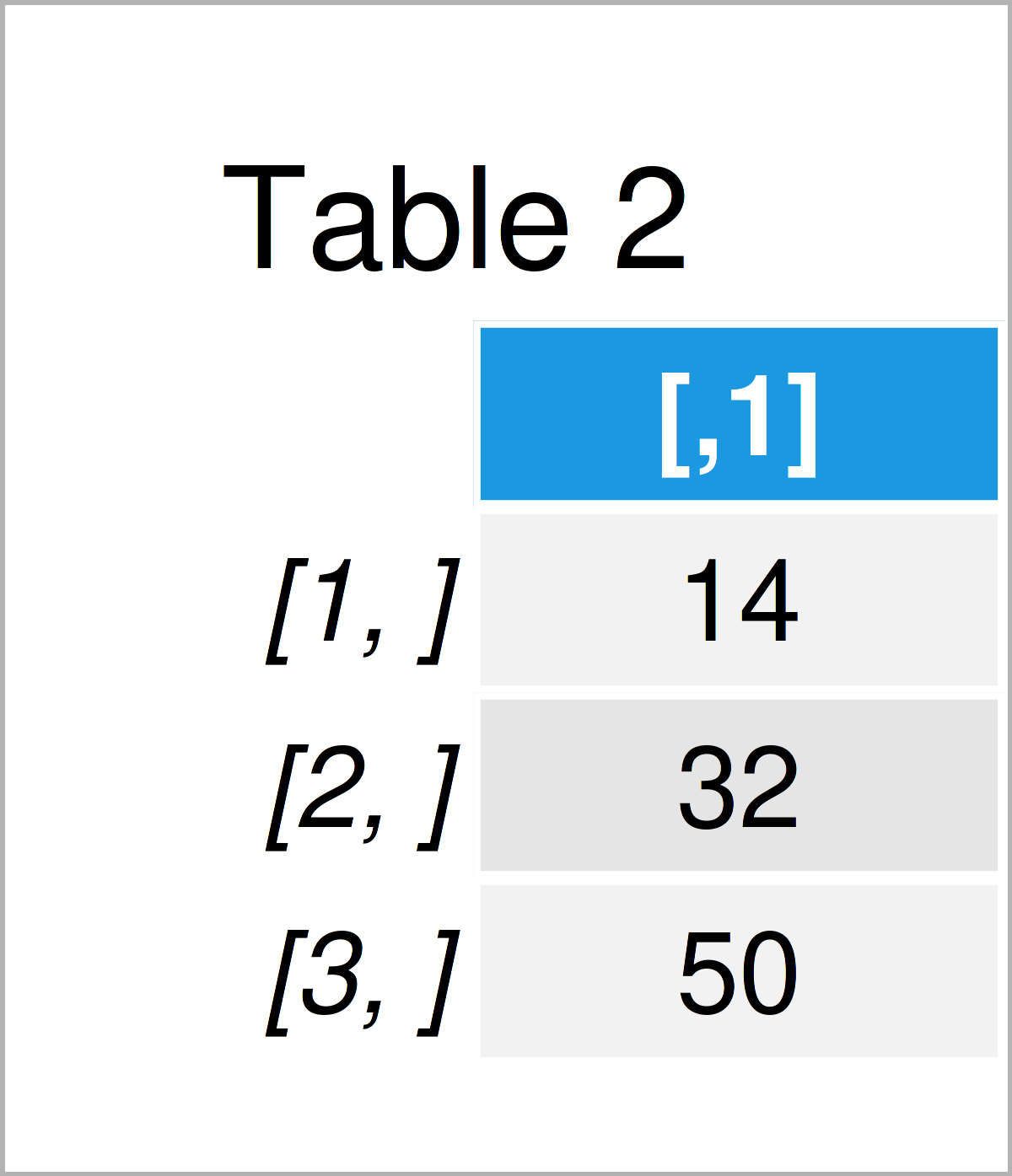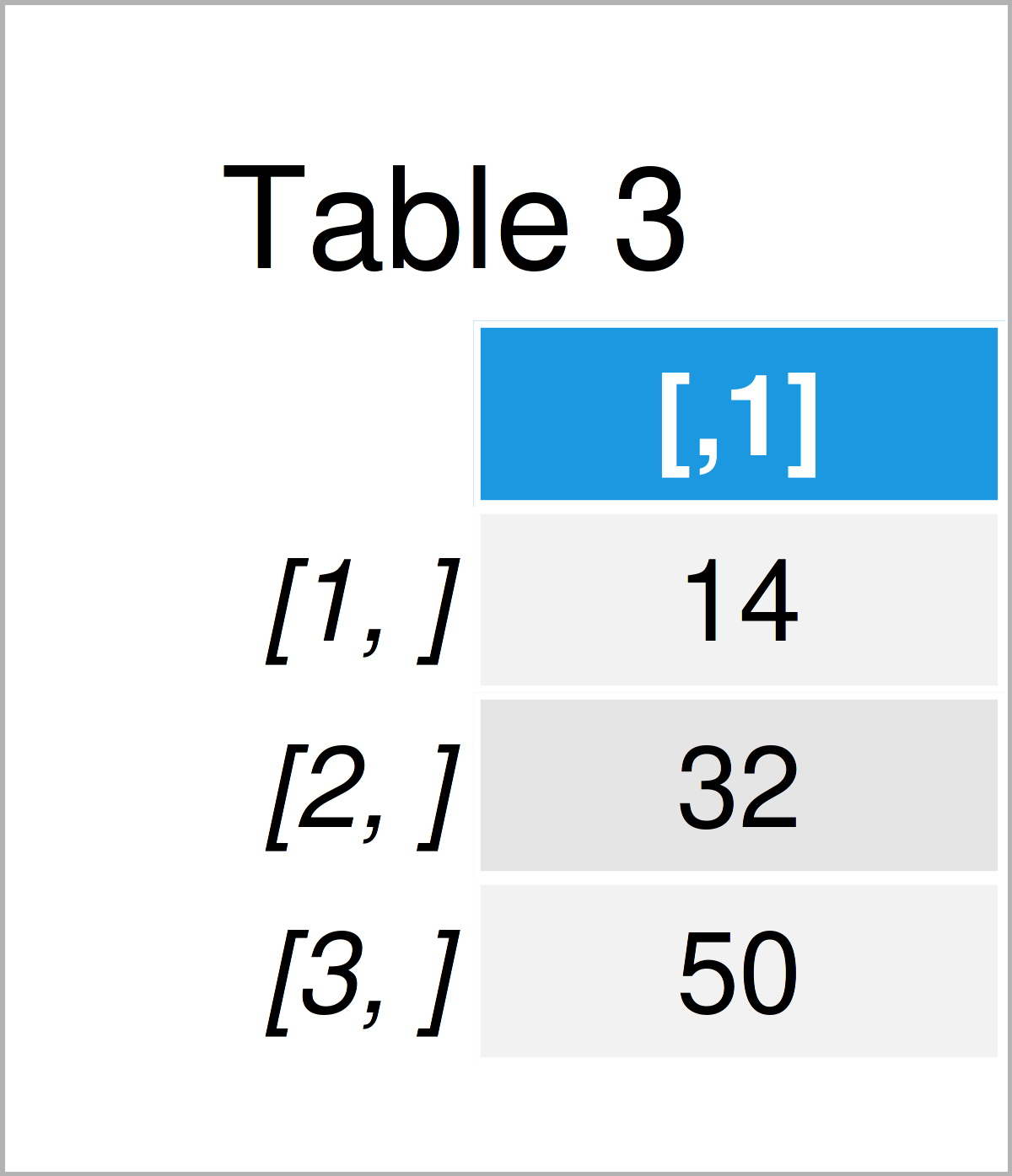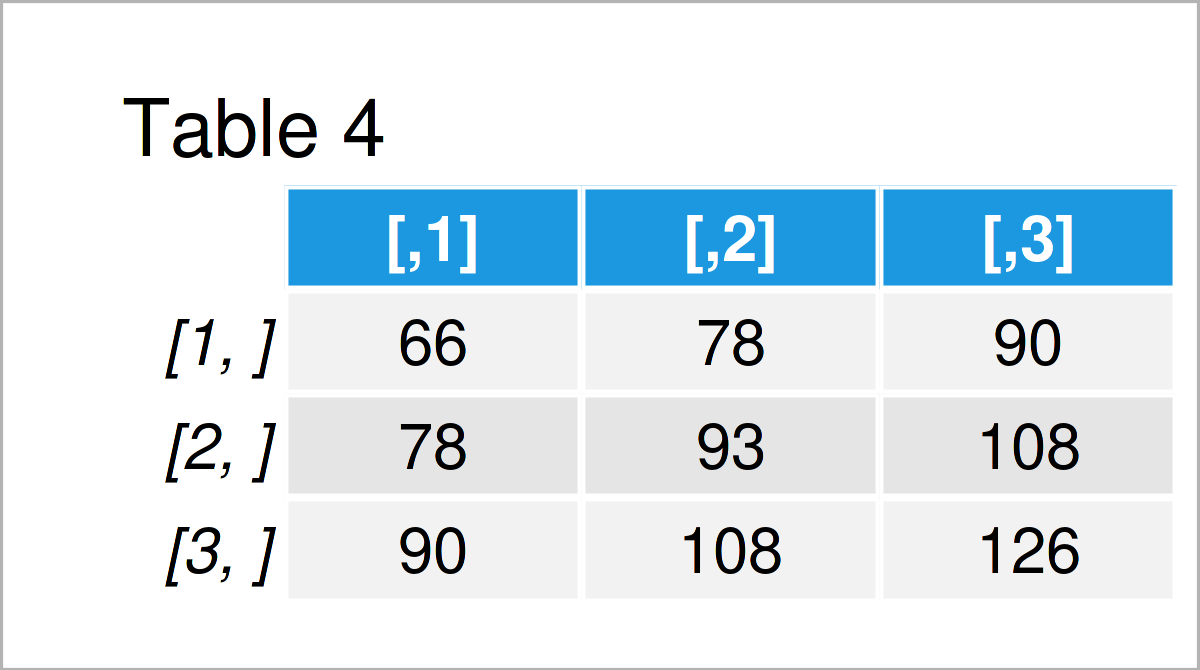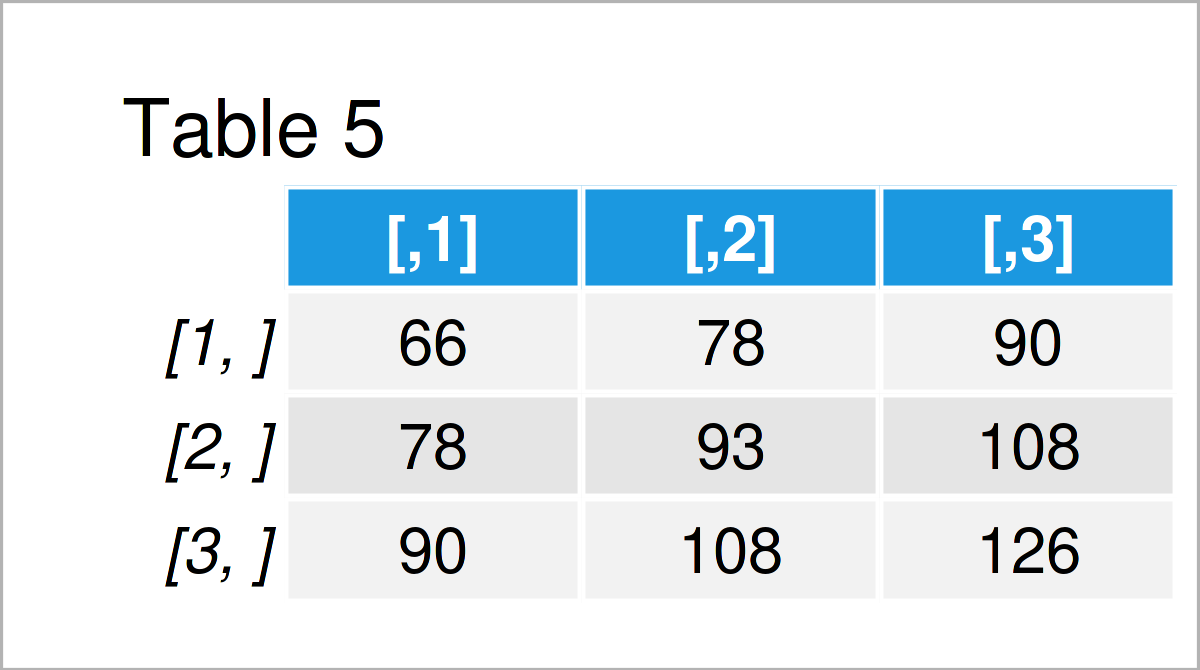# Matrix Cross Product in R (2 Examples) | crossprod & tcrossprod Functions

In this R tutorial you’ll learn how to calculate matrix cross products using the crossprod and tcrossprod functions.

The tutorial consists of these content blocks:

Here’s the step-by-step process…

## Creation of Example Data

The first step is to create some data that we can use in the examples later on:

```my_mat <- matrix(1:9, nrow = 3)               # Create example matrix
my_mat                                        # Print example matrix```Table 1 shows that our example data is composed of three rows and three columns.

Next, we also have to create an example vector:

```my_vec <- 1:3                                 # Create example vector
my_vec                                        # Print example vector
#  1 2 3```

Our vector contains three integers.

## Example 1: Cross Product Using crossprod() Function

In Example 1, I’ll explain how to calculate the cross product of a matrix and a vector.

For this, we can apply the crossprod function as shown in the following R code:

```my_crossprod1 <- crossprod(my_mat, my_vec)    # Apply crossprod function
my_crossprod1                                 # Print cross product```As shown in Table 2, the previous R programming code has created a new matrix object containing the cross product of our matrix and vector objects.

Note that the t function and %*% operator can also be used to compute a cross product matrix, but the crossprod function is usually faster and more efficient.

However, let’s compare the result of the t function and %*% operator to the crossprod function:

```my_crossprod2 <- t(my_mat) %*% my_vec         # t() function & %*% operator
my_crossprod2                                 # Print cross product```The output of the previous syntax is shown in Table 3: We have created exactly the same result as with the crossprod function.

## Example 2: Cross Product of Transpose of Matrix Using tcrossprod() Function

Similar to the crossprod function, we can apply the tcrossprod function to calculate the cross product of a transposed matrix.

Let’s assume that we want to calculate the cross product of the transpose of a matrix. Then, we can apply the code as shown below:

```my_tcrossprod1 <- tcrossprod(my_mat)          # Apply tcrossprod function
my_tcrossprod1                                # Print cross product of transpose of matrix```After executing the previous R programming code the matrix with the tcrossprod result shown in Table 4 has been created.

The tcrossprod function is also a faster alternative to the expression provided by the t function and %*% operator.

However, let’s use this alternative code as well:

```my_tcrossprod2 <- my_mat %*% t(my_mat)        # %*% operator & t() function
my_tcrossprod2                                # Print cross product of transpose of matrix```After running the previous R syntax the same matrix as after applying the tcrossprod command has been created.

## Video, Further Resources & Summary

In case you need further explanations on the examples of this page, you could have a look at the following video that I have published on my YouTube channel. In the video, I explain the R codes of this article in a live session.

In addition, you may want to read the other RStudio tutorials on my website.

Summary: This article has illustrated how to apply the crossprod and tcrossprod functions in R programming. If you have any further questions, don’t hesitate to let me know in the comments section.

Subscribe to the Statistics Globe Newsletter